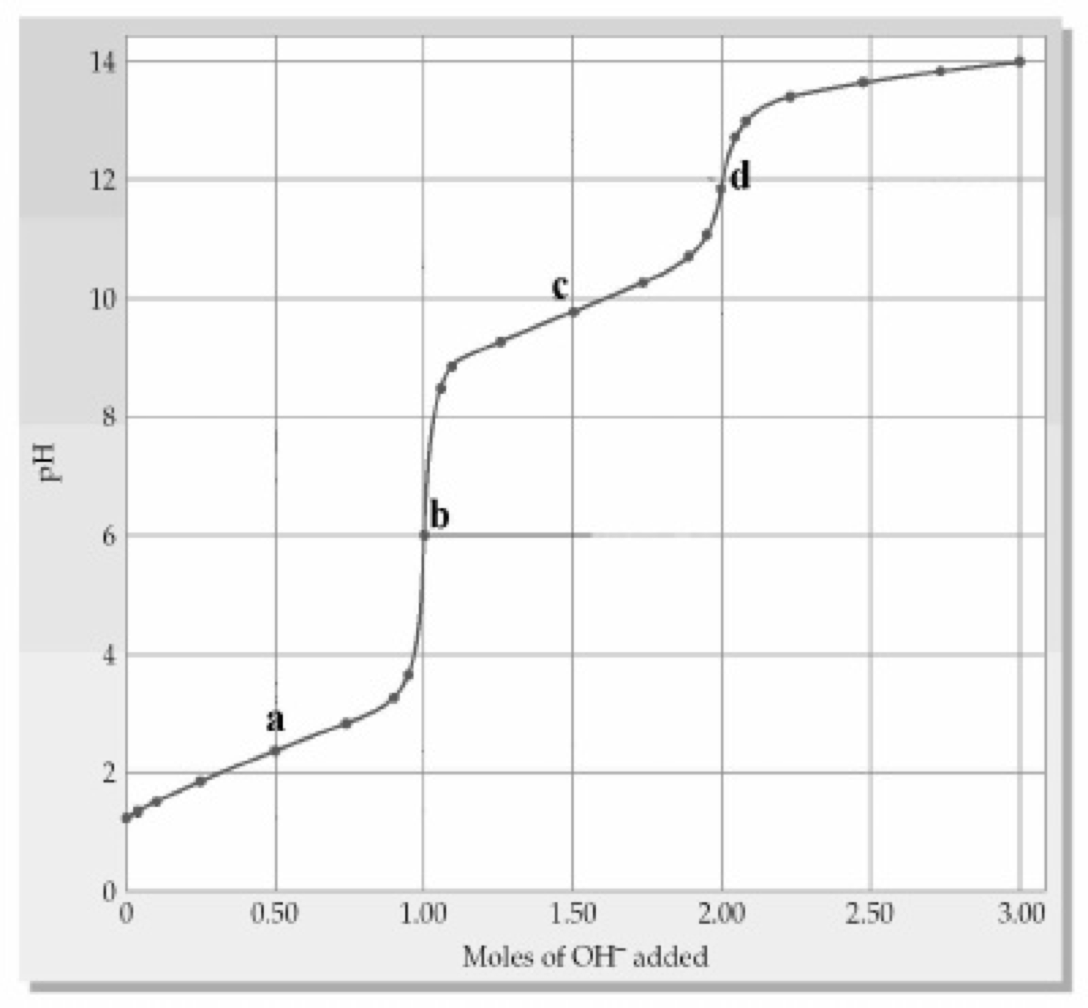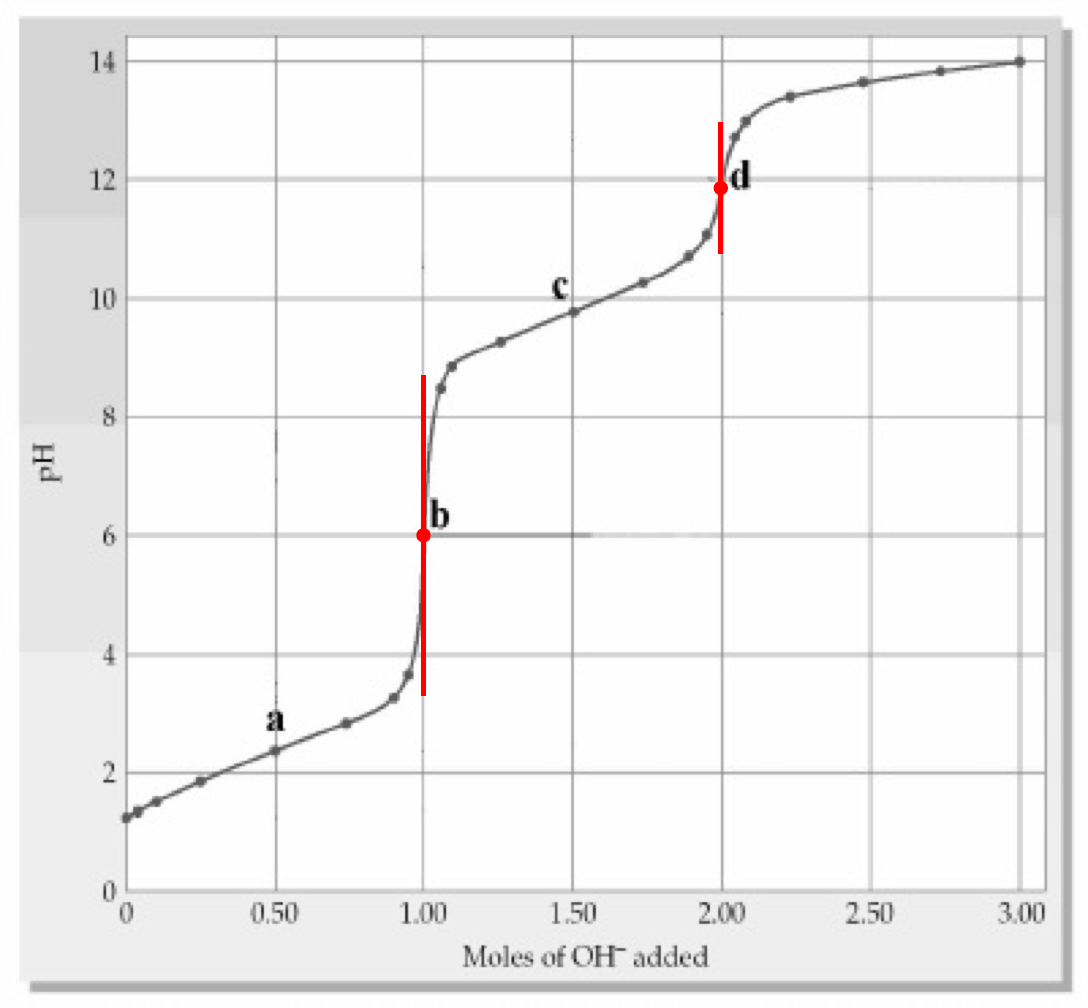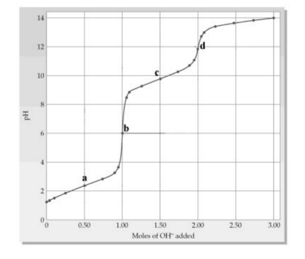# Problem: The following plot below shows a titration curve for the titration of 1.00 L of 1.00 M diprotic acid H2A with NaOH. Which point(s) a-d represent(s) the H 2X/HX - buffer region?(a) point a(b) point b(c) point c(d) point d(e) points a and c

🤓 Based on our data, we think this question is relevant for Professor O'Connor's class at CSU OHIO.

###### FREE Expert Solution

We’re being asked to determine which point(s) a–d represent(s) the H2X/HX– buffer region, given the following titration curve for the titration of 1.00 L of 1.00 M diprotic acid H2A with NaOH:Since H2A is a diprotic acid, this means it has two equivalence points

Recall that the equivalence point is the point in a titration curve where the pH increases abruptly. In the given titration curve, the two equivalence points are represented by points b and d:###### Problem Details

The following plot below shows a titration curve for the titration of 1.00 L of 1.00 M diprotic acid H2A with NaOH. Which point(s) a-d represent(s) the H 2X/HX - buffer region?

(a) point a

(b) point b

(c) point c

(d) point d

(e) points a and c